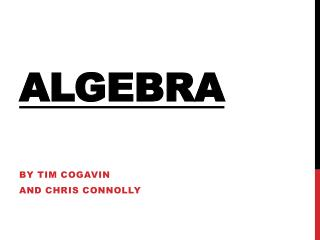DownloadDownload PresentationAlgebra

# Algebra

Télécharger la présentation## Algebra

- - - - - - - - - - - - - - - - - - - - - - - - - - - E N D - - - - - - - - - - - - - - - - - - - - - - - - - - -
##### Presentation Transcript

1. Algebra By Tim Cogavin And Chris Connolly

2. What Is Algebra? Algebra is when we replace numbers with letters to figure out an equation. Take this for example: I think of a number, add 8 to it and the result is 16 Obviously the number is 8. But we can write it out like this… x+8=16

3. However algebra has been used for many more complicated things. Did you know that we use algebra for everything from our gaming to nuclear warheads?

4. Two of the most famous mathematicians are Albert Einstein and Hamilton. We have all our gaming and television systems thanks to this equation……. i₂=j₂=k₂=ijk=-1 This equation was used to animate 3D objects. Therefore giving us our video games and movies

5. However the most famous mathematic equation is… e=mc₂ This equation was used for nuclear warheads during the war This equation was invented by Albert Einstein

6. Thanks For watching!